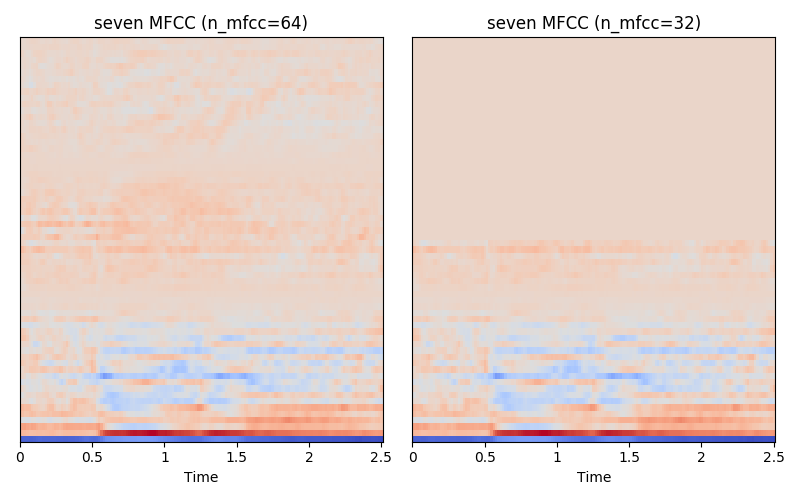# Understanding common features used : [Audio/Speech Processing Part 1]

If you’re interested to work on digital audio processing, in particular, speech processing, this is the article for you. Here I will talk about the common feature used for various tasks such as speech-to-text, text-to-speech, noise-cancellations.

This is Part 1 of the the series:

# Contents:

1. Waveforms
2. Spectrogram
3. Mel-spectrograms
4. Mel Frequency Cepstral Coefficients (MFCC)

All of the following plots can be reproduced here.

# Waveforms

This is how the number ‘seven’ look like, in a 1D representation, called a waveform. But what do this actually mean? To really understand, we look at how frequencies are represented on a fundamental level.

Consider these two frequencies, 1Hz and 2Hz, when we add them together, they are considered a superposition of these two frequencies.

Now we look back at the number ‘seven’ spoken by a human, it is superposition of many frequencies, that a human can speak and hear (20Hz —20kHz).

Now, how do we actually really pinpoint what are the frequencies? For that we look at a 2D representation of an audio: spectrogram.

# Spectrogram

By performing Fourier Transform on the subframes of the waveform, we get its frequency representation on the y-axis. Here we limit to 8kHz, because the audio is in 16kHz. The redder the values, means there are greater amplitude of such frequencies in that time period.

Notice there are red values in the top left hand corner. This is because the ‘shhh’ sound in the earliert part of the word ‘seven’ is of higher frequency (4kHz-8kHz), hence the redder values in that region.

On the other hand, there are more of ‘vibrational’ frequencies observed in the middle part, and this is caused by vibration of our vocal tract, in the later half of the word ‘seven’.

To understand this physically, simply touch your adam’s apple, and say the word ‘SHHH-EEEE-VEEEEN’ really slowly, and realise that at the ‘SHHH’ part, your vocal tracts are not vibrating at all.

# Mel-spectrogram

Another interesting commonly used feature is the Mel filterbank.

The reason this is studied is because, there is a nonlinear relationship as the frequencies increases. That is, the difference between 10Hz to 20Hz is very perceptible, but the difference between 10010Hz to 10020Hz is almost non-perceptible.

On the left are figures demonstrating how the audio experts consider the relationship should be like.

Using this relationship, we transform the spectrogram of ‘seven’ to its mel filterbank. Above is a side-by-side comparison of the linear spectrogram, and its mel filterbank conversion. Notice that the the higher frequencies are now ‘squeezed’ together, while that of the lower frequencies are spread further apart.

Note that this conversion is also loosely considered to be a dimensionality-reduction technique as the number of frequency bins reduced from 256 frequency bins to 64 mel bins.

# MFCC

A further post-processed feature of the mel filterbank is the Mel-Frequency Cepstral Coefficients (MFCC). It is constructed by performing dicrete cosine transform (DCT) on each frame of the log mel-spectrogram. Most of the time, we remove the higher coefficients, as they are mostly redundant.Left : All 64 coefficients of the MFCC. Right : Only keep the lower 32 coefficients.

Here we have two MFCCs, on the left, the MFCC has all 64 coefficients, on the right, we removed the higher 32 coefficients. As observed, we can’t really interpret what this MFCC as well as we could for the previously observed spectrograms, as the DCT causes decorrelation between adjacent frames. Now, how do we do we interpret the reduced dimensionality?